## Multistrain models

### How to interpret changing trends?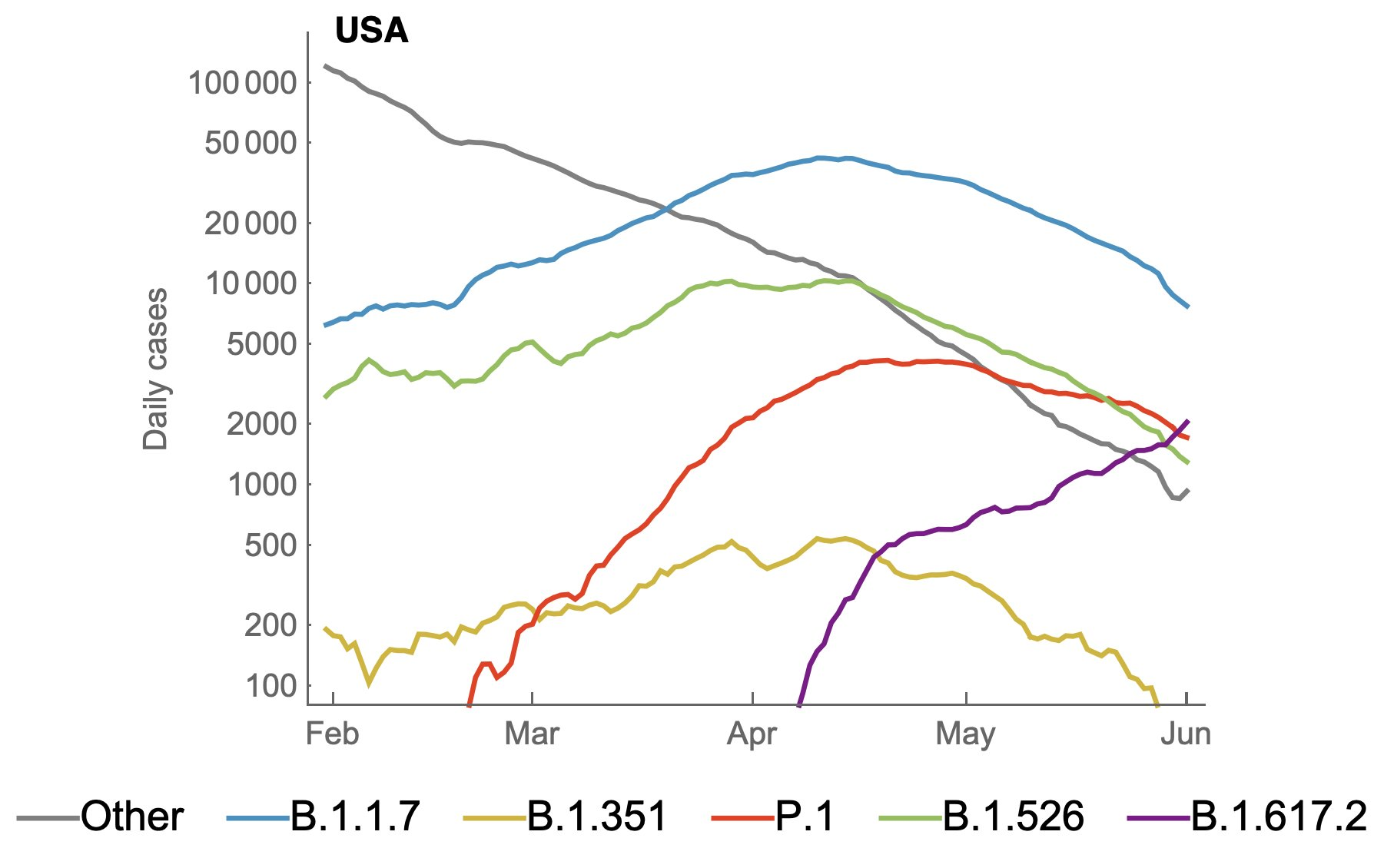### Should we see antigenic change? Turnover? Coexistence?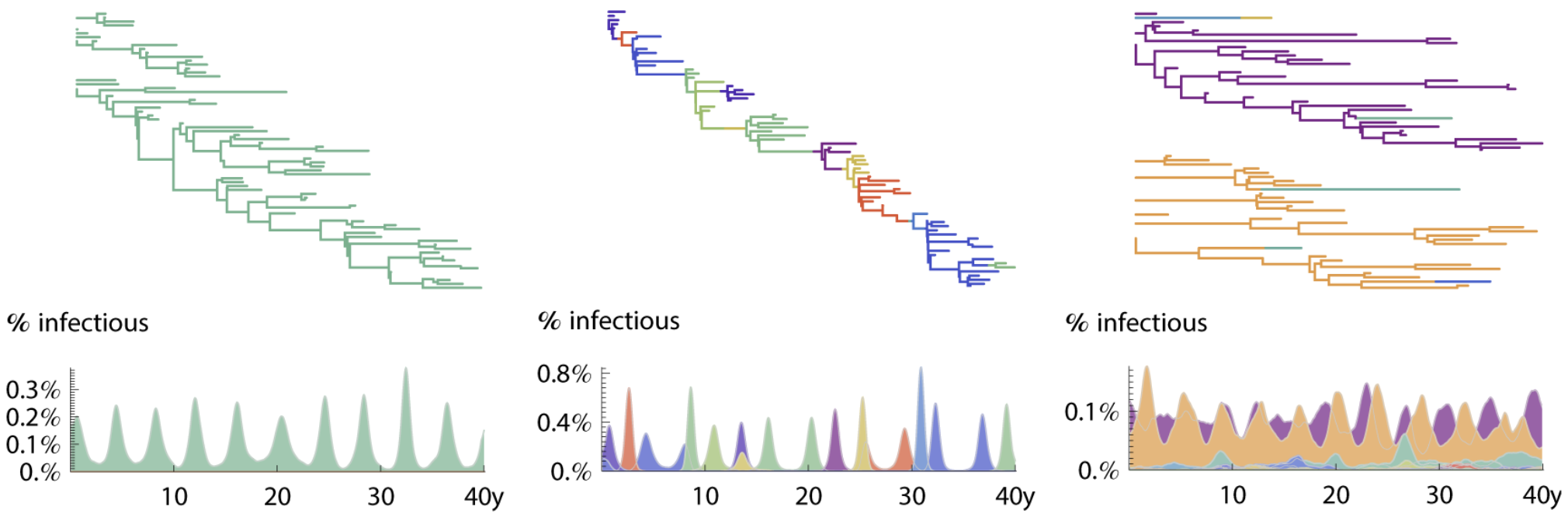### Resistance to vaccines? Antimicrobials?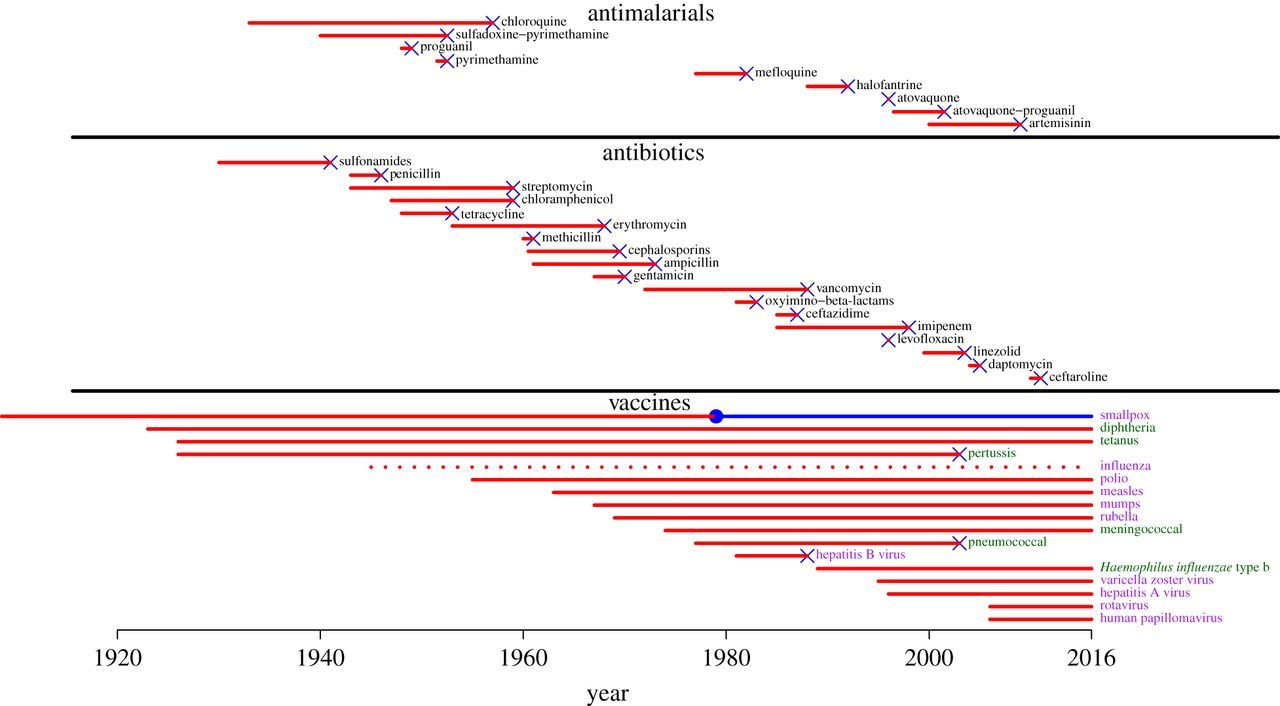### Model types are fuzzy

Statistical

Mechanistic (mathematical v. agent-based)

### History-based models

Track individuals' histories of infection, $S_{(0)}, S_{(1)}, S_{(1,2)},...$

Cross-immunity reduces probability of infection or infectiousness

Numerically unwieldy: $2^n$ histories for $n$ strains

### Dimensional reduction

Requires cross-immunity through reduced transmission (only)

Cross-immunity must be in context of antigenic "neighborhoods"

(with $n$ strains in $m$ neighborhoods, system is $n$ x $(m+1)$)

Impossible to calculate immunity to new strains

### Status-based models

Track individuals S, I, or R to each strain

$O(n)$ equations possible

"Polarized" immunity: If $i$ and $j$ cross-react, infection with $j$ leads to perfect immunity to $i$ in some fraction of hosts

### A "simple" status-based model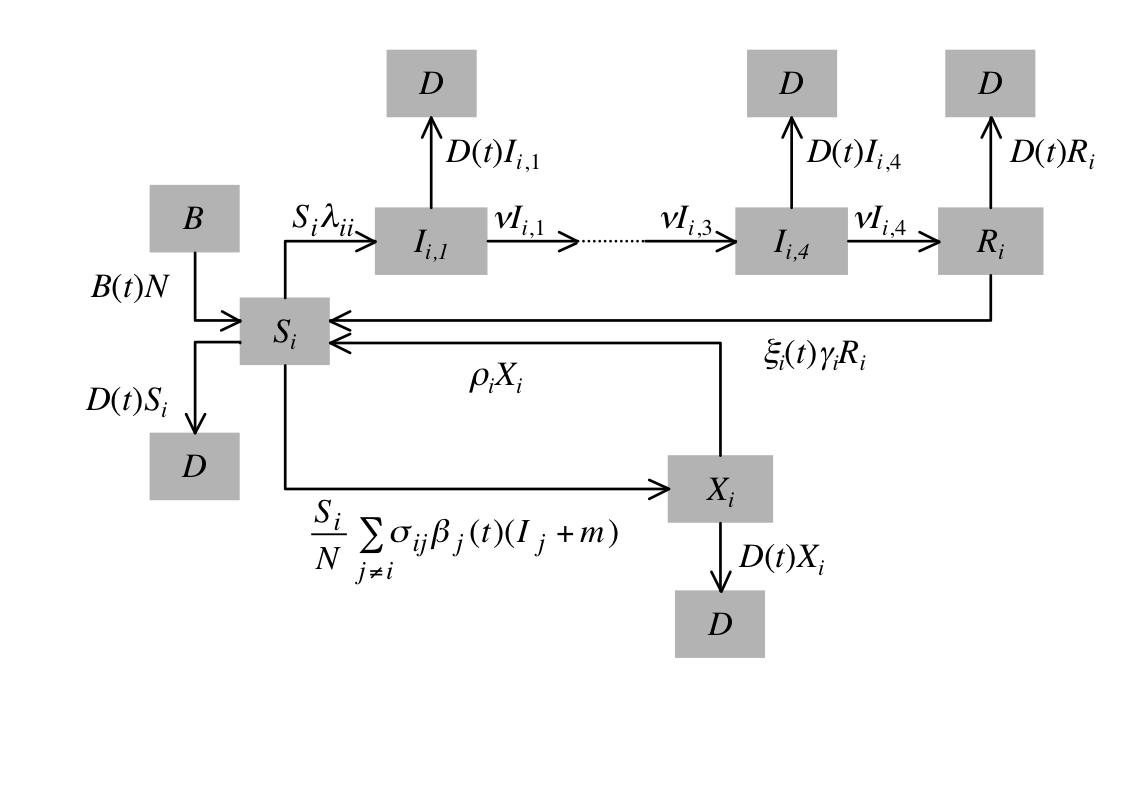### Implicit assumption

Proportion of population not infected by second strain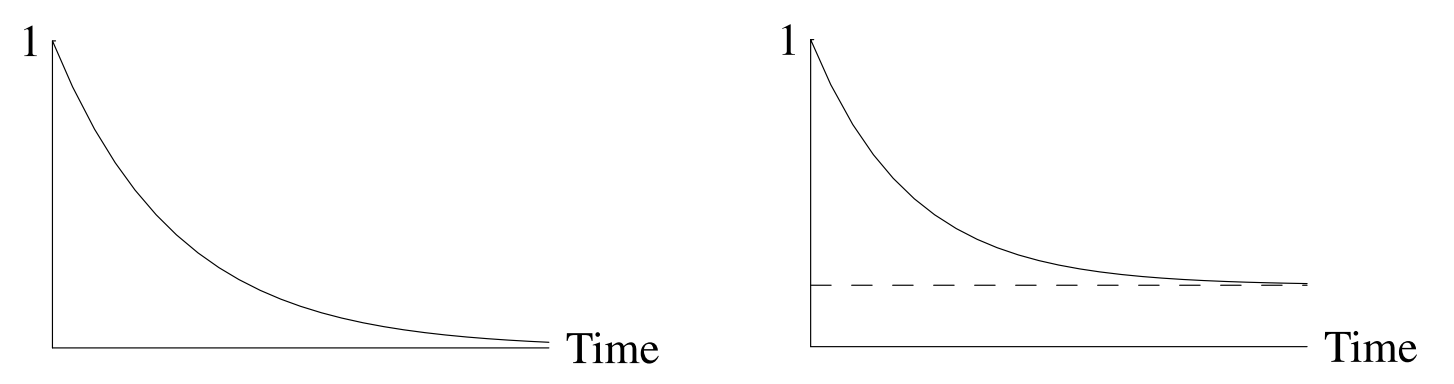### Some options for multistrain models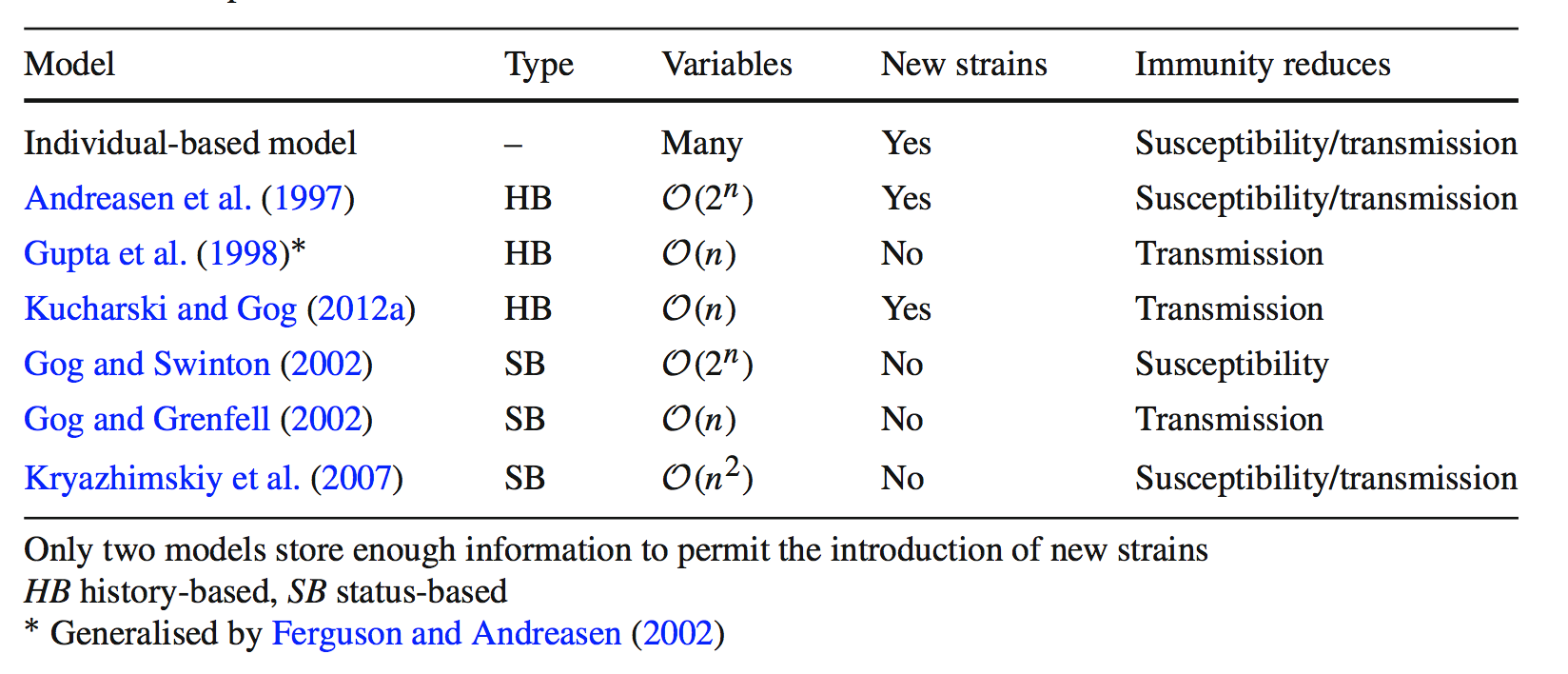### Classic problem: invasion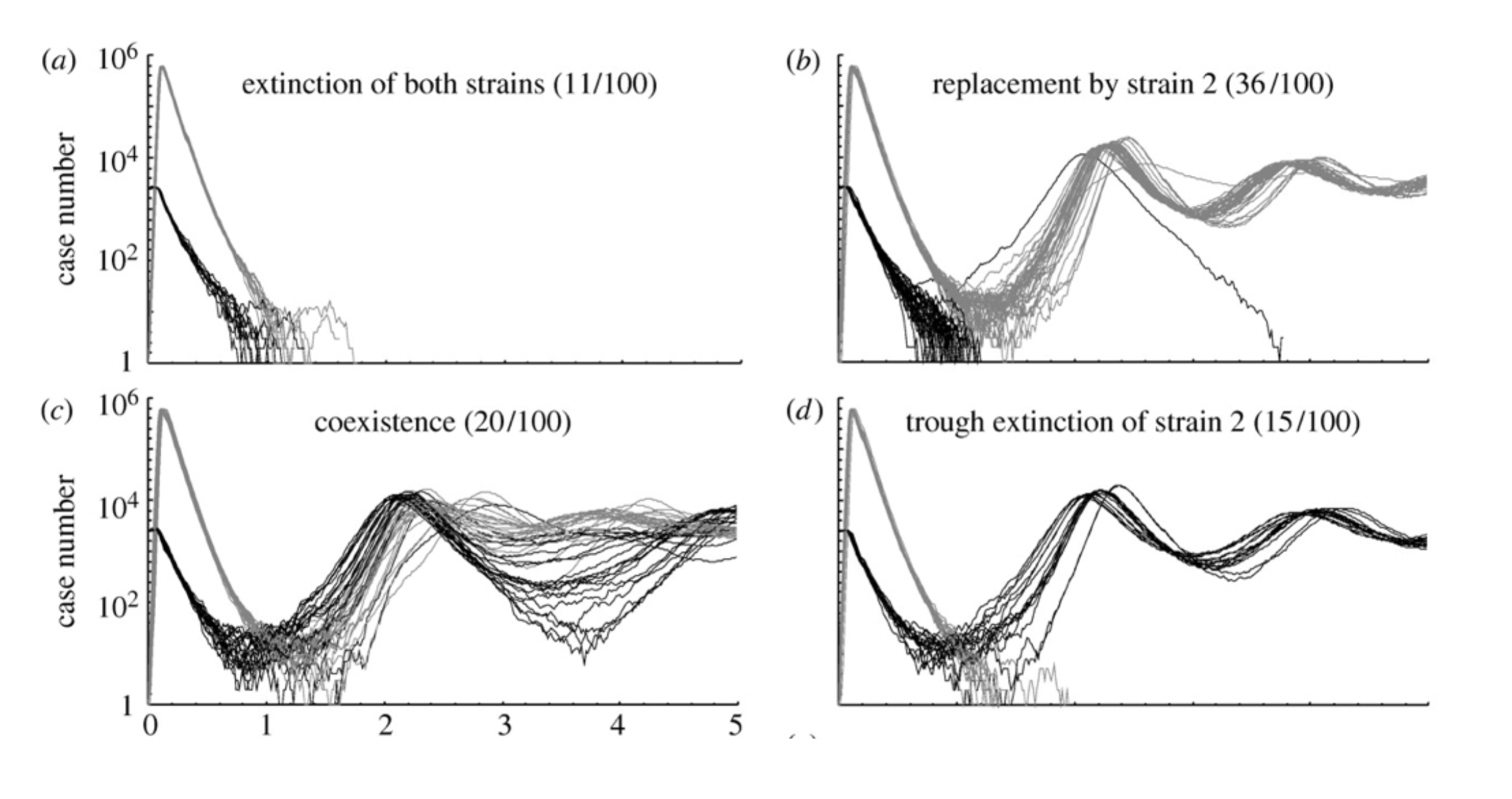### Subtle differences change dynamics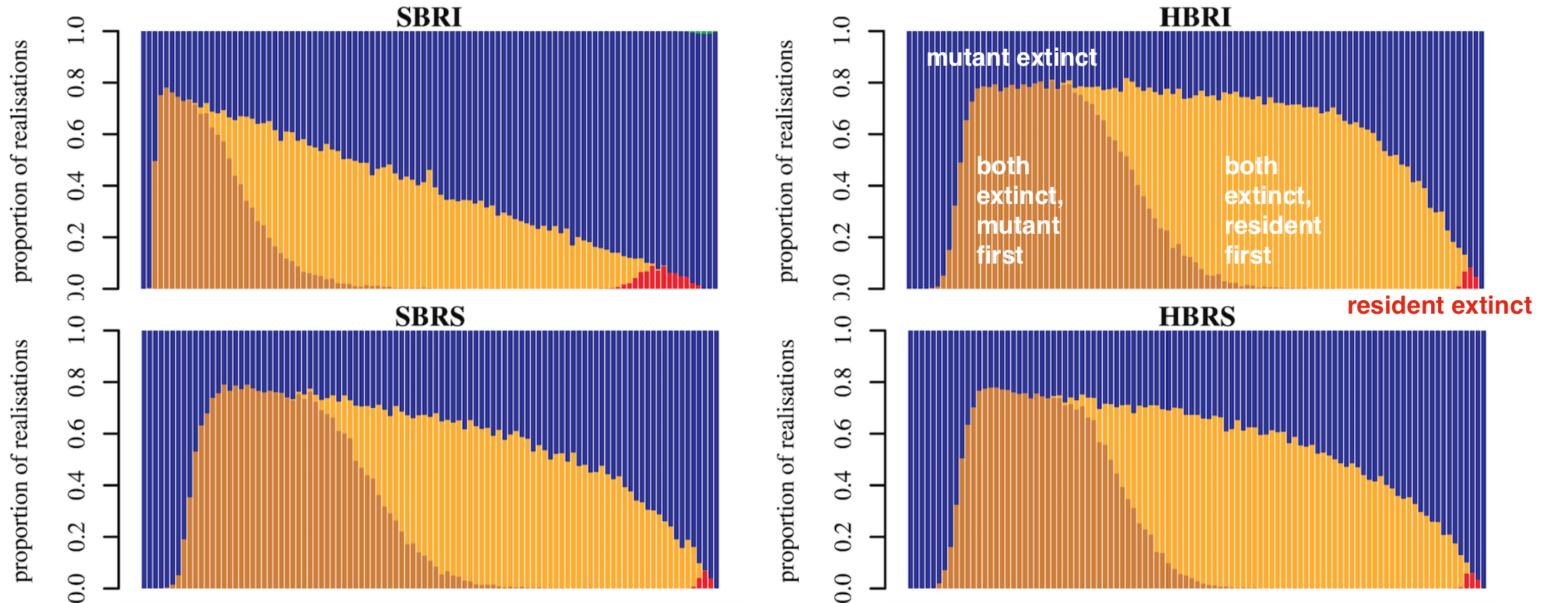Cross-immunity

Which model promotes coexistence?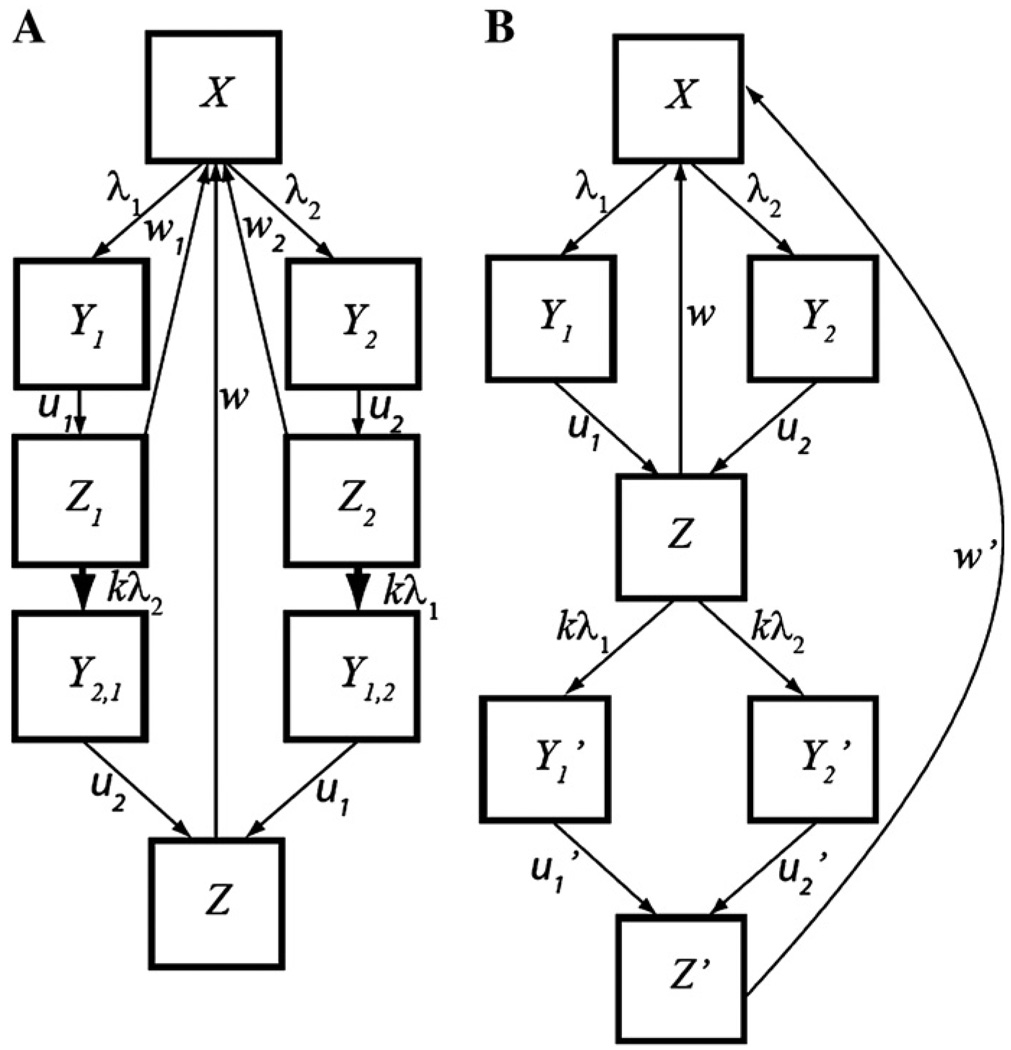### Model A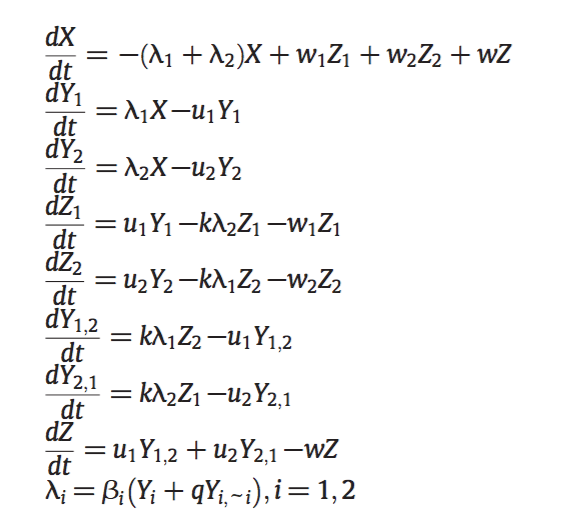### Model B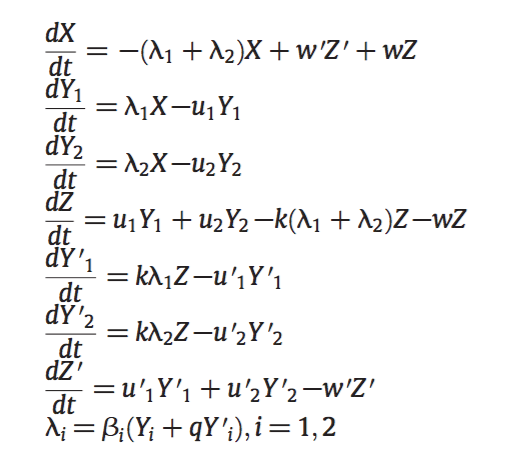### Does this model bias for coexistence?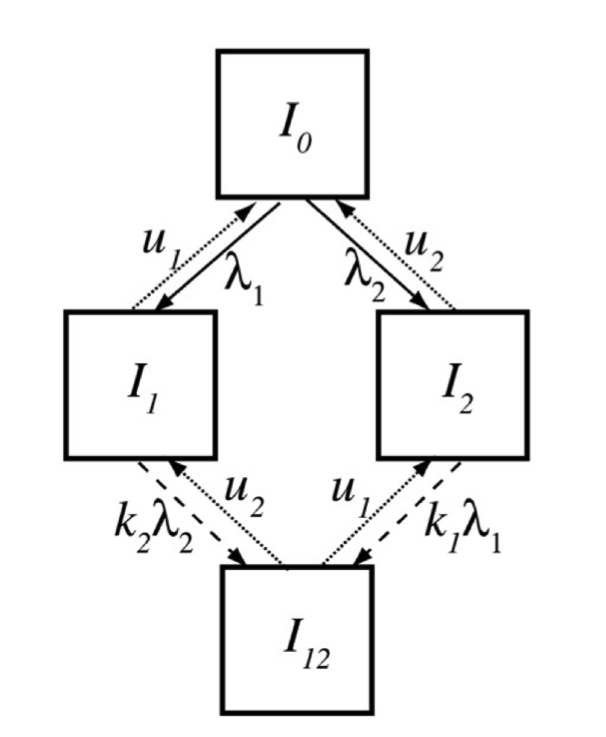### Equations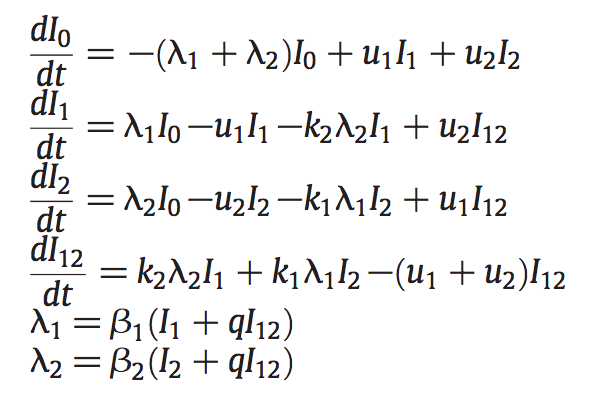### Equations

Ecological dynamics depend on strain composition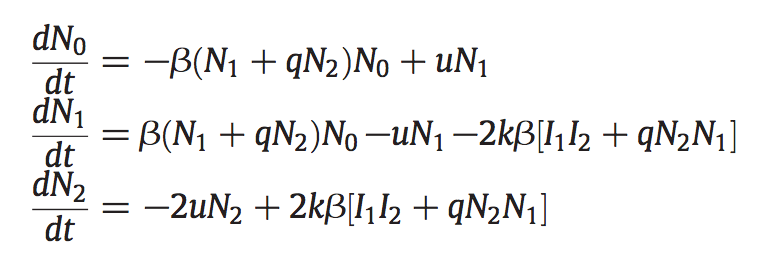### Multiplicity of infection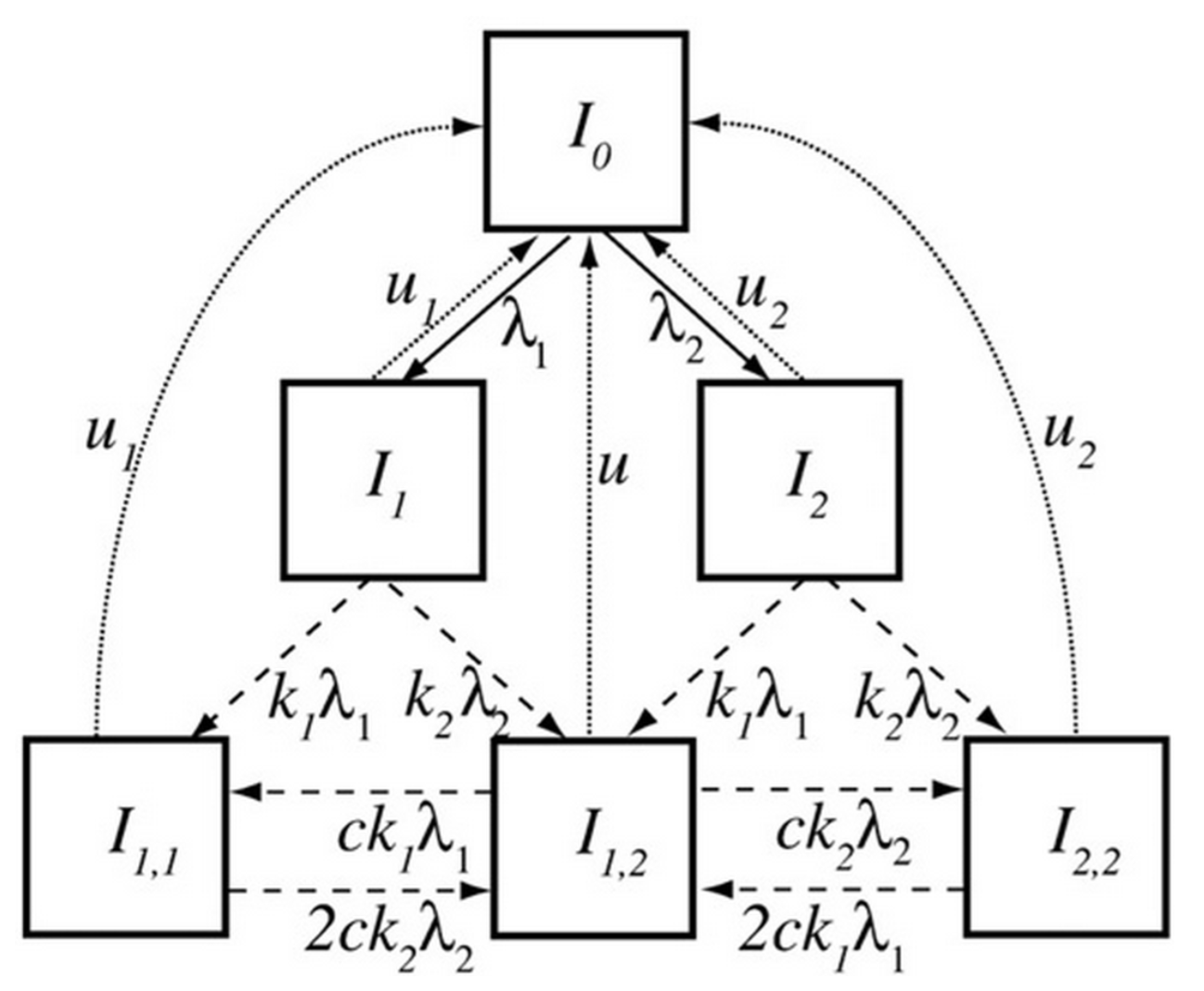### ODEs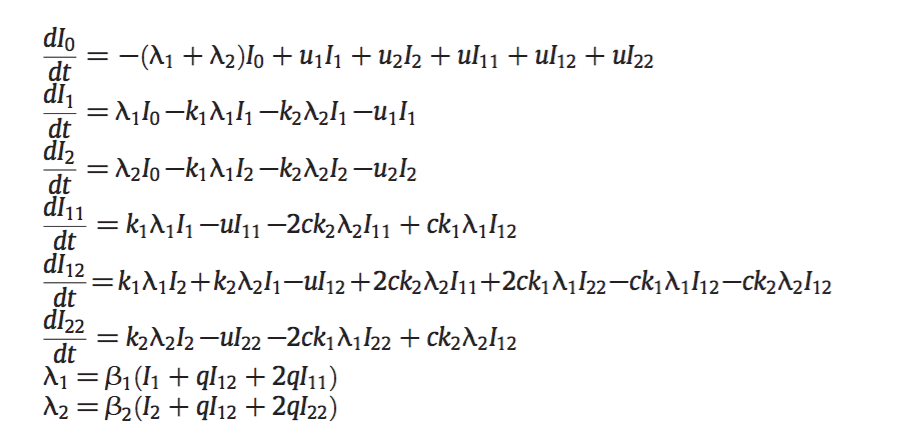### Ecological dynamics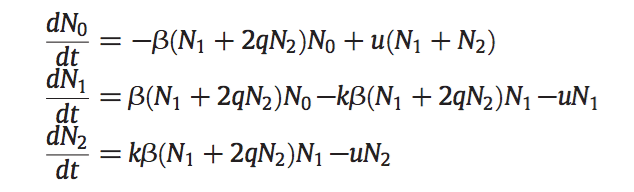### Ancestor tracing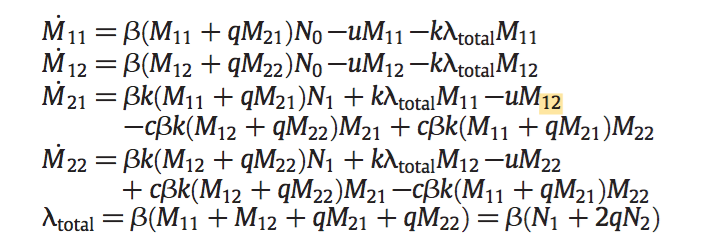### The question of strain space

Continuous or discrete?

How many dimensions?

### Continuous space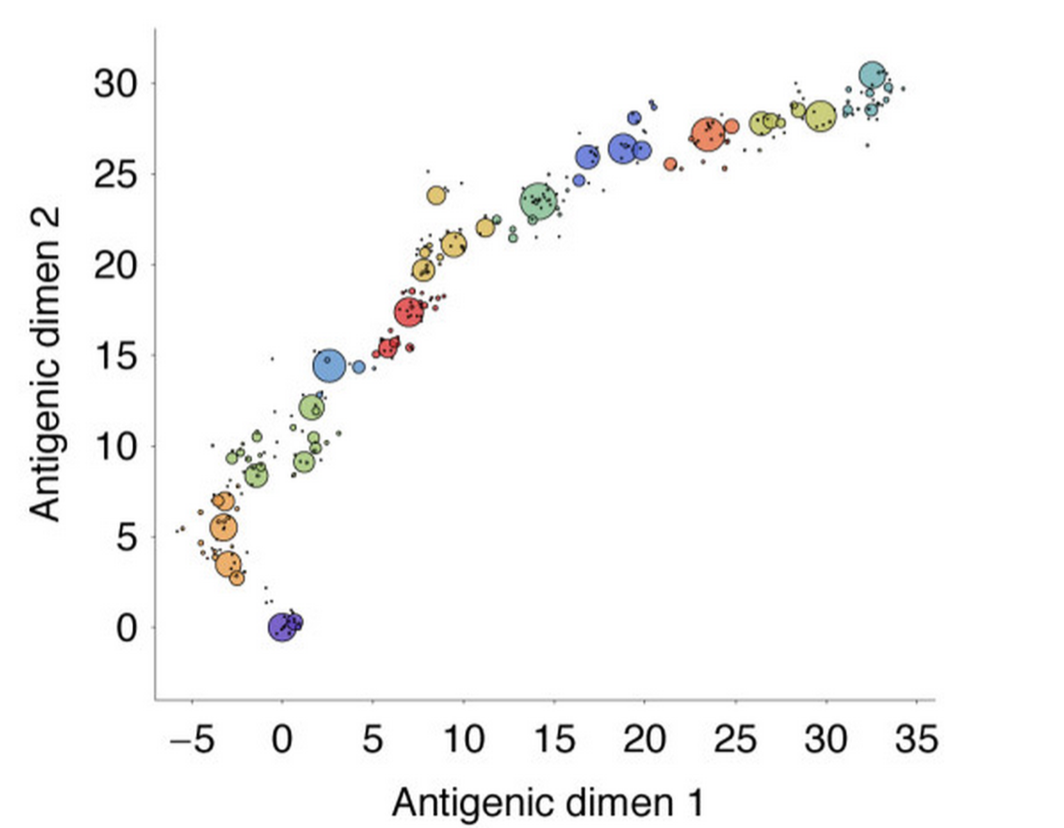### Discrete space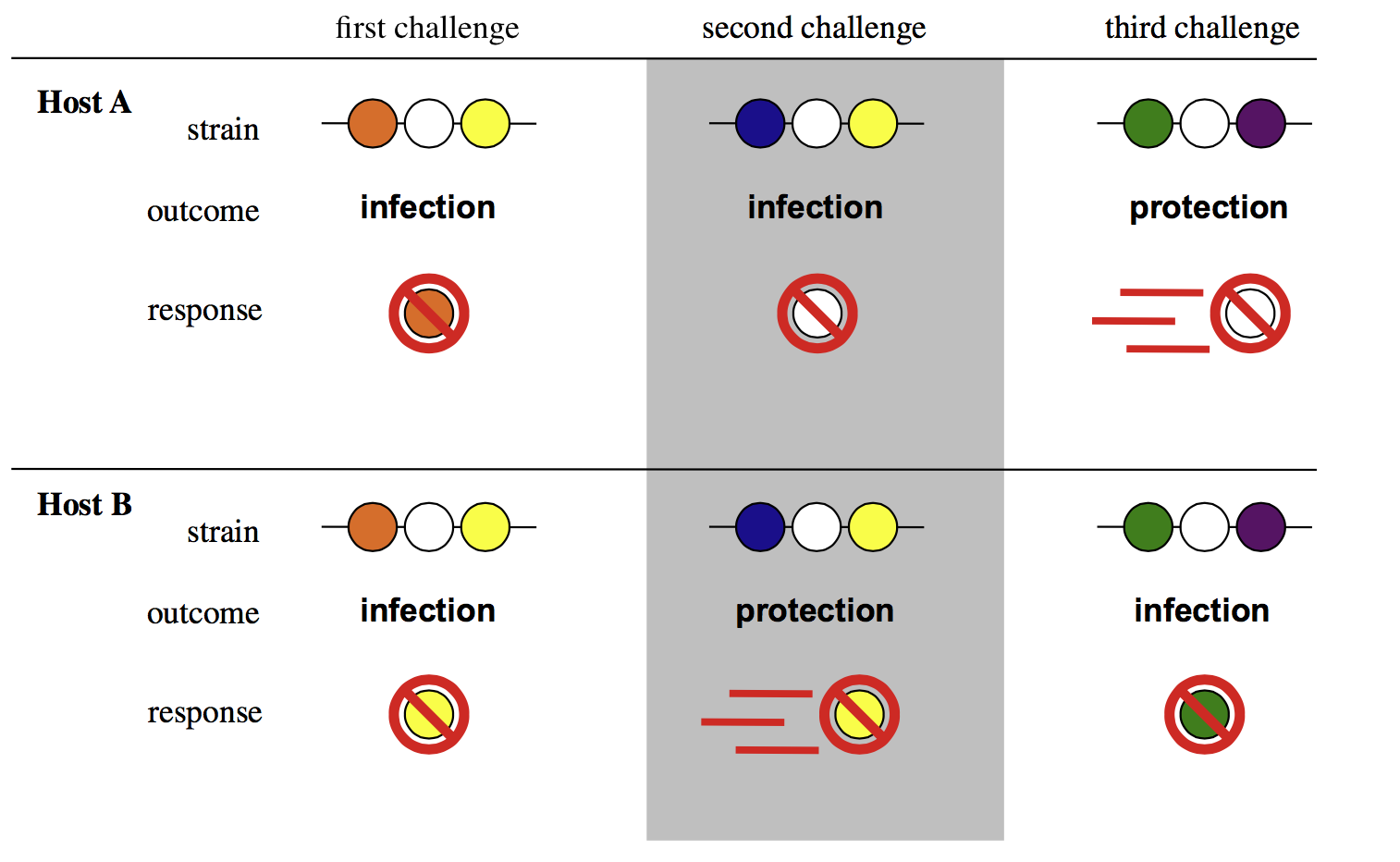#### Immunodominance and breadth affect diversity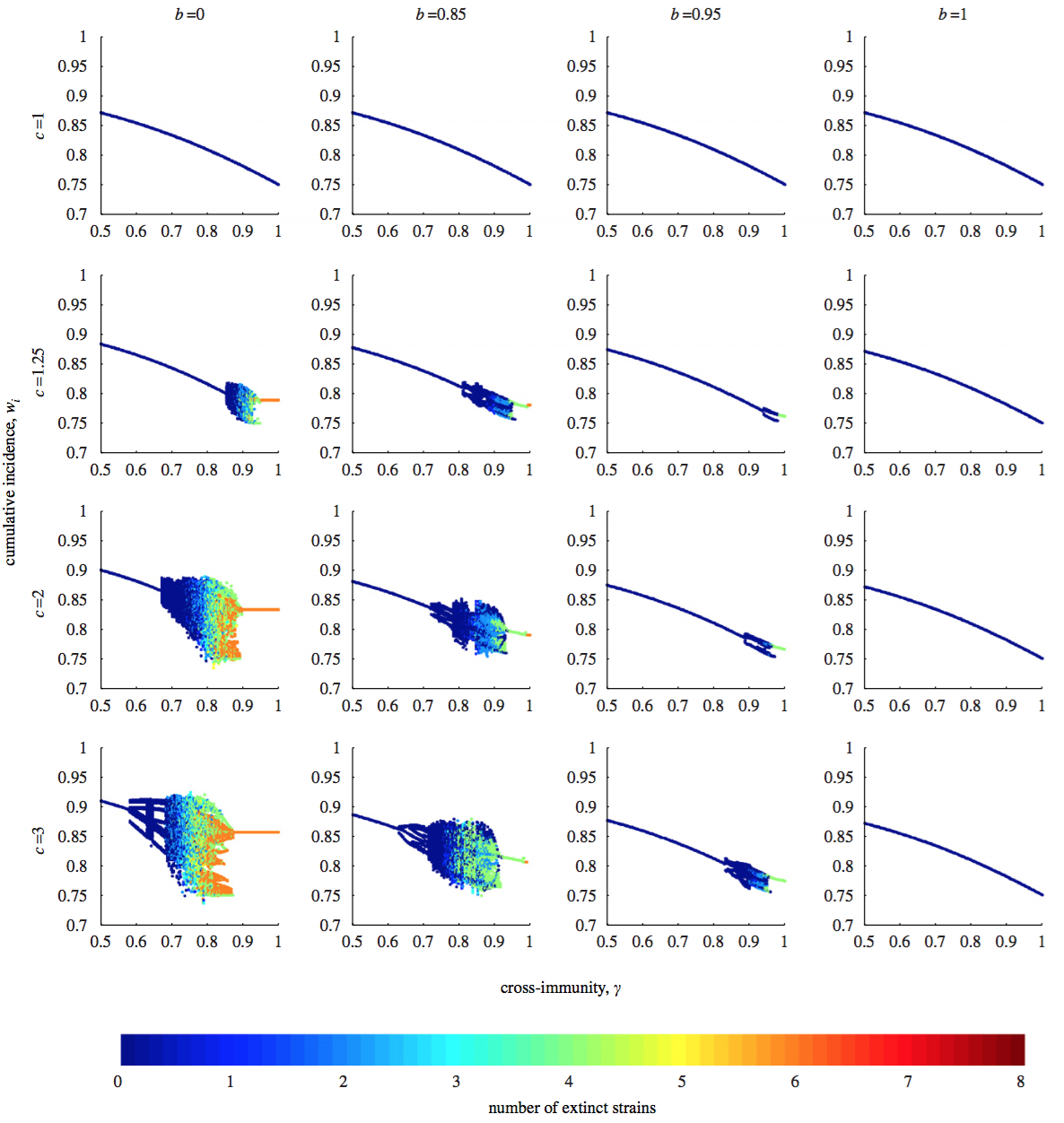### Agent-based models

Potentially most efficient with many strains

Support complex interactions

Naturally incorporate demographic stochasticity

### Analytic approaches

Equilibria: Solve for $S_{i}'=I_{i}'=...=0$

Stability analysis: Linearize at equilibria

Invasion analysis: Is $I_{2}'>0$ at $I_{1}^*$?

Integration: Calculate $I_{1}(t), I_{2}(t),...$

### Numerical integration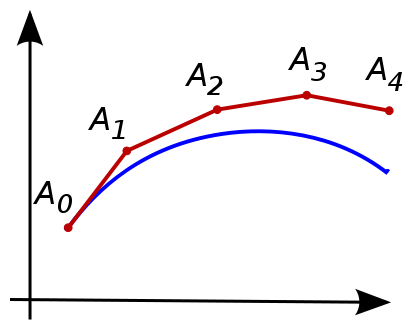Approximate solution to $N'=f(N)$:

$$N(t_0+\Delta t) \approx N_0+ f(N_0) \Delta t = N_1$$

Re-iterating, we get

$$N_{z+1} \approx N_{z}+f(N_{z}) \Delta t$$

$$N_{z+1} \approx N_{z}+f(N_{z}) \Delta t$$

We could assume $f(x)$ is constant for $\Delta t$ (Euler's method)

Even better, average over multiple points!

### A second-order method

$$N_{z+1} \approx N_{z}+f(N_{z}) \Delta t$$

$$M_{z+1}=N_z+f(N_{z}) \Delta t$$

$$N_{z+1}=N_z+\frac{1}{2}\left[ f(N_{z})+f(M_{z+1})\right] \Delta t$$

### 4th-order Runge-Kutta

$$k_1=f(N_z) \Delta t$$

$$k_2=f\left(N_z+\frac{1}{2}k_1 \right) \Delta t$$

$$k_3=f\left(N_z+\frac{1}{2}k_2 \right) \Delta t$$

$$k_4=f\left(N_z+k_3 \right) \Delta t$$

$$N_{z+1}=N_z+\frac{1}{6}(k_1+2k_2+2k_3+k_4)$$

### Considerations for multistrain models

Form of immunity (duration, strength, polarity)

Effect of immunity (susceptibility, infectiousness, clearance)

Coinfections and timing of interactions

Accuracy for individuals v. populations

Dimensionality and discretization of strain space

Analytic and numeric approaches, including stochasticity